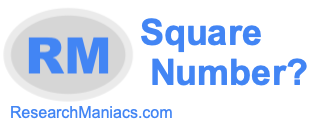Is 202 a square number?Here we will answer the question: "Is 202 a square number?" You could also ask "Is 202 a perfect square?" Square number and perfect square mean the same thing.

Anyway, for 202 to be a square number, 202 must be the product of an integer multiplied by itself. In other words, the square root of 202 must equal a whole number (integer).

There is no integer that you can multiply by itself that will make 202. Furthermore, the square root of 202 is not an integer. Thus, 202 is NOT a square number.

The answer to the question: "Is 202 a square number?" is No.
The answer to the question: "Is 202 a perfect square?" is also No.

Square Number?
Enter another number to see if it is a square number:

Is 203 a square number?
Find out if the next number on our list is a perfect square.